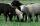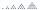Gasoline

35 l of gasoline is to be divided into four canisters so that in the third canister was 5 l less than the first canister, the fourth canister 10 l more than in the third canister and the second canister half what in the first canister. How many liters of fuel are in each canisters?

Result

a =  10 l
b =  5 l
c =  5 l
d =  15 l

Solution:

a+b+c+d=35
c= a-5
d = 10+c
b = a/2

a+b+c+d = 35
a-c = 5
c-d = -10
a-2b = 0

a = 10
b = 5
c = 5
d = 15

Calculated by our linear equations calculator.

Leave us a comment of this math problem and its solution (i.e. if it is still somewhat unclear...):Be the first to comment!To solve this verbal math problem are needed these knowledge from mathematics:

Do you have a system of equations and looking for calculator system of linear equations? Tip: Our volume units converter will help you with the conversion of volume units.

Next similar math problems:

1. Gasoline canisters35 liters of gasoline is to be divided into 4 canisters so that in the third canister will have 5 liters less than in the first canister, the fourth canister 10 liters more than the third canister and the second canister half of that in the first canist
2. Lord RamWhen lord Ram founded the breed white sheep was 8 more than black. Currently white sheep are four times higher than at the beginning and black three times more than at the beginning. White sheep is now 42 more than the black. How many white and black sh
3. Factory and divisionsThe factory consists of three auxiliary divisions total 2,406 employees. The second division has 76 employees less than 1st division and 3rd division has 212 employees more than the 2nd. How many employees has each division?
4. Theorem proveWe want to prove the sentence: If the natural number n is divisible by six, then n is divisible by three. From what assumption we started?
5. Three workshopsThere are 2743 people working in three workshops. In the second workshop works 140 people more than in the first and in third works 4.2 times more than the second one. How many people work in each workshop?
6. Three numbersWhat is three numbers which have the property: the sum of the reciprocals of the first and second numbers is 12/7, the first and third 11/24 and the second and the third 3/8.
7. Linsys2Solve two equations with two unknowns: 400x+120y=147.2 350x+200y=144
8. Elimination methodSolve system of linear equations by elimination method: 5/2x + 3/5y= 4/15 1/2x + 2/5y= 2/15
9. LegsCancer has 5 pairs of legs. The insect has 6 legs. 60 animals have a total of 500 legs. How much more are cancers than insects?
10. Three unknownsSolve the system of linear equations with three unknowns: A + B + C = 14 B - A - C = 4 2A - B + C = 0
11. BottlesThe must is sold in 5-liter and 2-liter bottles. Mr Kucera bought a total of 216 liters in 60 bottles. How many liters did Mr. Kucera buy in five-liter bottles?
12. AntennasIf you give me two antennas will be same. If you give me again your two antenna I have a 5× so many than you. How many antennas have both mans?
13. CandiesIf Alena give Lenka 3 candy will still have 1 more candy. If Lenka give Alena 1 candy Alena will hame twice more than Lenka. How many candies have each of them?
14. Two equationsSolve equations (use adding and subtracting of linear equations): -4x+11y=5 6x-11y=-5
15. Lee isLee is 8 years more than twice Park's age, 4 years ago, Lee was three times as old. How old was Lee 4 years ago?
16. A fishermanA fisherman buys carnivores to fish. He could buy either 6 larvae and 4 worms for \$ 132 or 4 larvae and 7 worms per \$ 127. What is the price of larvae and worms? Argue the answer.
17. Linear systemSolve a set of two equations of two unknowns: 1.5x+1.2y=0.6 0.8x-0.2y=2